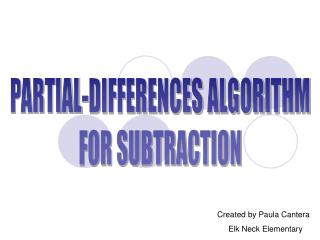DownloadDownload PresentationPARTIAL-DIFFERENCES ALGORITHM FOR SUBTRACTION

# PARTIAL-DIFFERENCES ALGORITHM FOR SUBTRACTION

Télécharger la présentation## PARTIAL-DIFFERENCES ALGORITHM FOR SUBTRACTION

- - - - - - - - - - - - - - - - - - - - - - - - - - - E N D - - - - - - - - - - - - - - - - - - - - - - - - - - -
##### Presentation Transcript

1. PARTIAL-DIFFERENCES ALGORITHM FOR SUBTRACTION Created by Paula Cantera Elk Neck Elementary

2. When using this algorithm, you will first calculate partial differences, subtracting from left to right, one place-value column at a time. Next, you will add all the partial differences to find the total difference.

3. Let’s review some vocabulary: 456 - 342 minuend subtrahend 114 difference

4. ***HELPFUL HINT*** When first starting to use this algorithm, it may help to write the minuend and subtrahend out in expanded form before you begin. This step can be eliminated as you become more comfortable with the algorithm.

5. Let’s give one a try!

6. Let’s write the minuend and subtrahend in expanded form. 496 400 + 90 + 6 - 231 200 + 30 + 1

7. Remember--- subtract from left to right, one place-value at a time. 496 400 + 90 + 6 - 231 + 1 200 + 30

8. 496 400 + 90 + 6 - 231 + 1 200 + 30 -200) 200 Subtract the hundreds. (400

9. 496 400 + 90 + 6 - 231 + 1 200 + 30 -200) 200 Subtract the hundreds. (400 60 (90 - 30) Subtract the tens.

10. 496 400 + 90 + 6 - 231 + 1 200 + 30 -200) 200 Subtract the hundreds. (400 60 Subtract the tens. (90 - 30) 5 (6 - 1) Subtract the ones.

11. 496 400 + 90 + 6 - 231 + 1 200 + 30 -200) 200 Subtract the hundreds. (400 60 Subtract the tens. (90 - 30) + 5 (6 - 1) Subtract the ones. 265 Now, add the partial sums. (200+60+5)

12. Let’s try another one! A little bit harder this time!

13. 7,513 7,000 + 500 + 10 + 3 - 2,442 2,000 + 400 + 40 + 2 -2000) 5,000 Subtract the thousands. (7,000

14. (500 7,513 7,000 + 500 + 10 + 3 - 2,442 2,000 + 400 + 40 + 2 - 2000) 5,000 (7,000 Subtract the thousands. 100 - 400) Subtract the hundreds.

15. - 30 7,513 7,000 + 500 + 10 + 3 - 2,442 2,000 + 400 + 40 + 2 - 2000) 5,000 (7,000 Subtract the thousands. 100 (500 - 400) Subtract the hundreds. (10 - 40) Subtract the tens. Note the negative symbol!! Since you normally would regroup in this instance, you will record the partial difference as a negative number and subtract it rather than add it when adding up your partial differences.

16. - 30 7,513 7,000 + 500 + 10 + 3 - 2,442 2,000 + 400 + 40 + 2 - 2000) 5,000 (7,000 Subtract the thousands. 100 (500 - 400) Subtract the hundreds. (10 - 40) Subtract the tens. 1 Subtract the ones. (3 - 2)

17. - 30 7,513 7,000 + 500 + 10 + 3 - 2,442 2,000 + 400 + 40 + 2 - 2000) 5,000 (7,000 Subtract the thousands. 100 (500 - 400) Subtract the hundreds. (10 - 40) Subtract the tens. + 1 Subtract the ones. (3 - 2) 5,071 Now, add the partial sums. (5,000 + 100 - 30+ 1) * Remember the negative symbol

18. Partial differences are much easier to work with and you will feel empowered when you discover that, with practice, you just might be able to use this algorithm to subtract numbers mentally!

19. Give the Partial-Differences Algorithm a try. You might find that it makes subtraction of mult-digit numbers easier for you!